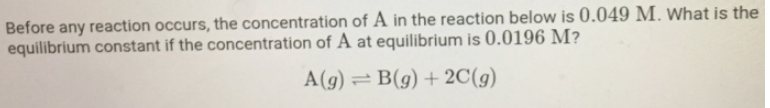# Problem: Before any reaction occurs, the concentration of A in the reaction below is 0.049 M. What is the equilibrium constant if the concentration of A at equilibrium is 0.0196 M? A(g) ⇌ B (g) + 2C (g)

###### FREE Expert Solution
82% (481 ratings)###### Problem Details

Before any reaction occurs, the concentration of A in the reaction below is 0.049 M. What is the equilibrium constant if the concentration of A at equilibrium is 0.0196 M?

A(g) ⇌ B (g) + 2C (g)